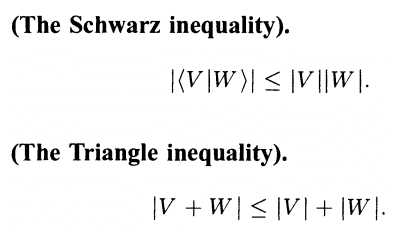# Schwarz inequality

## Homework Statement

For x,y,z ## \in \mathbb {R^+} ##, prove that
## \sqrt {x (3 x +y) } + \sqrt {y (3y +z) } + \sqrt {z(3z +x)} \leq ~ 2(x +y+ z) ##

## Homework Equations## The Attempt at a Solution

I don't know which inequality among the above two has to be applied.
I am trying to solve it by inspection. I don't know the standard approach to solve it.